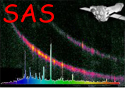XMM-Newton Science Analysis System

ommosaic (ommosaic-2.11) [xmmsas_20211130_0941-20.0.0]

## Cross-correlation

The cross-correlation coefficient, Rbetween 2 images,, with offsetsbetween their x and y origins, respectively, is computed from

R,

where,,,

where nx and ny are the image dimensions in the x and y directions, respectively. In the above formulae it is assumed that the mean image values have been subtracted from the images. R can vary from -1 to +1.

XMM-Newton SOC -- 2021-11-30